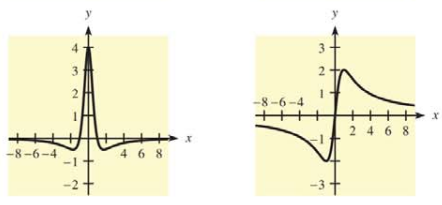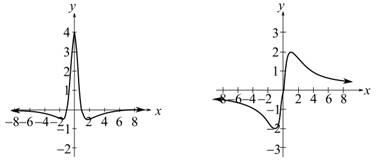Chapter 10.1, Problem 48E### Mathematical Applications for the ...

11th Edition
Ronald J. Harshbarger + 1 other
ISBN: 9781305108042

#### Solutions

Chapter
Section### Mathematical Applications for the ...

11th Edition
Ronald J. Harshbarger + 1 other
ISBN: 9781305108042
Textbook Problem

# In Problems 47 and 48, two graphs are given. One is the graph of f , and the other is the graph of f Decide which is which and explain your reasoning.To determine

The graph of the function f(x) and the graph of the derivative f'(x) which are the two graphs are given and explain the answer.Explanation

Given Information:

The provided graphs of f(x) and f(x) are as follows:

Explanation:

Consider the provided graphs,

For function f which is differentiable in interval (a,b),

If f(x)>0 then function f is increasing in that interval and if f(x)<0 then function f is decreasing in that interval

### Still sussing out bartleby?

Check out a sample textbook solution.

See a sample solution

#### The Solution to Your Study Problems

Bartleby provides explanations to thousands of textbook problems written by our experts, many with advanced degrees!

Get Started

#### In Exercises 1124, find the indicated limits, if they exist. 24. limxx2x+1

Applied Calculus for the Managerial, Life, and Social Sciences: A Brief Approach

#### True or false? det(A) is a number, not a matrix.

Precalculus: Mathematics for Calculus (Standalone Book)

#### True or False: is a convergent series.

Study Guide for Stewart's Single Variable Calculus: Early Transcendentals, 8th

#### Explain the role of voluntary participation in informed consent.

Research Methods for the Behavioral Sciences (MindTap Course List)